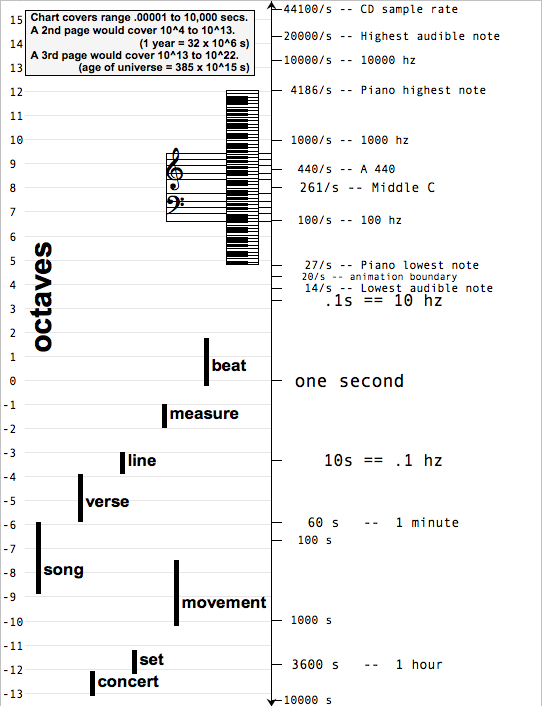### Frequency Range

This is a vertical number line, labelled in seconds, showing event durations.  At the bottom are long-lasting musical patterns (sets, concerts); at the top are quick events-- also called notes.

The chart title could be "Duration of events which, if repeated, humans can distinguish".

We're aware of lots of repeating patterns: with 100s of repeats per second as notes; per minute as rhythm; per hour as verse.

The left column is octaves (frequency doublings) from 1 per second.

Fun fact: the range shown is almost the same as the Richter scale, as 33 octaves = 10 Richters.   (10 octaves = 2^10 = 1024 ~ 1000 = 10^3 = 3 Richters)

Also:  this chart covers the range (10^-5) seconds to (10^4)s.

A 2nd page would cover 10^4 to 10^13.  A year is 32x10^6 seconds.

A 3rd page would cover 10^13 to 10^22.  The age of the universe is 385x10^15 seconds.